# Sine, Cosine and Tangent in Four Quadrants

## Sine, Cosine and Tangent

The three main functions in trigonometry are Sine, Cosine and Tangent.They are easy to calculate:

Divide the length of one side of a
right angled triangle by another side

... but we must know which sides!

For an angle θ, the functions are calculated this way:

 Sine Function: sin(θ) = Opposite / Hypotenuse Cosine Function: cos(θ) = Adjacent / Hypotenuse Tangent Function: tan(θ) = Opposite / Adjacent

### Example: What is the sine of 35°?Using this triangle (lengths are only to one decimal place): sin(35°) = Opposite / Hypotenuse = 2.8/4.9 = 0.57...

## Cartesian Coordinates

Using Cartesian Coordinates we mark a point on a graph by how far along and how far up it is:The point (12,5) is 12 units along, and 5 units up.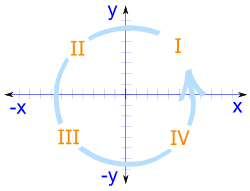When we include negative values, the x and y axes divide the space up into 4 pieces:

Quadrants I, II, III and IV

(They are numbered in a counter-clockwise direction)

• In Quadrant I both x and y are positive,
• in Quadrant II x is negative (y is still positive),
• in Quadrant III both x and y are negative, and
• in Quadrant IV x is positive again, and y is negative.

Like this: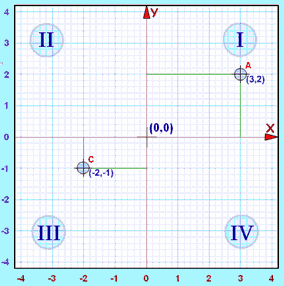(horizontal)
Y
(vertical)
Example
I Positive Positive (3,2)
II Negative Positive
III Negative Negative (−2,−1)
IV Positive Negative

Example: The point "C" (−2,−1) is 2 units along in the negative direction, and 1 unit down (i.e. negative direction).

Both x and y are negative, so that point is in "Quadrant III"

## Sine, Cosine and Tangent in the Four Quadrants

Now let us look at what happens when we place a 30° triangle in each of the 4 Quadrants.

In Quadrant I everything is normal, and Sine, Cosine and Tangent are all positive:

### Example: The sine, cosine and tangent of 30°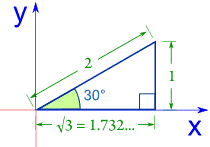Sine sin(30°) = 1 / 2 = 0.5 Cosine cos(30°) = 1.732 / 2 = 0.866 Tangent tan(30°) = 1 / 1.732 = 0.577

But in Quadrant II, the x direction is negative, and both cosine and tangent become negative:

### Example: The sine, cosine and tangent of 150°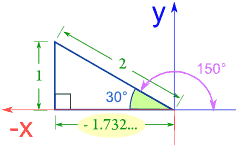Sine sin(150°) = 1 / 2 = 0.5 Cosine cos(150°) = −1.732 / 2 = −0.866 Tangent tan(150°) = 1 / −1.732 = −0.577

In Quadrant III, sine and cosine are negative:

### Example: The sine, cosine and tangent of 210°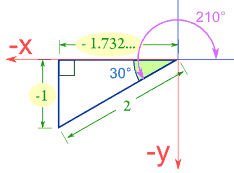Sine sin(210°) = −1 / 2 = −0.5 Cosine cos(210°) = −1.732 / 2 = −0.866 Tangent tan(210°) = −1 / −1.732 = 0.577

Note: Tangent is positive because dividing a negative by a negative gives a positive.

In Quadrant IV, sine and tangent are negative:

### Example: The sine, cosine and tangent of 330°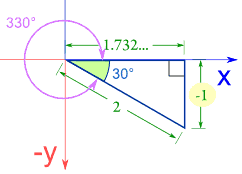Sine sin(330°) = −1 / 2 = −0.5 Cosine cos(330°) = 1.732 / 2 = 0.866 Tangent tan(330°) = −1 / 1.732 = −0.577

There is a pattern! Look at when Sine Cosine and Tangent are positive ...

• All three of them are positive in Quadrant I
• Sine only is positive in Quadrant II
• Tangent only is positive in Quadrant III
• Cosine only is positive in Quadrant IV

This can be shown even easier by: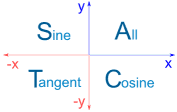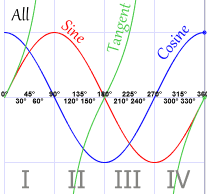This graph shows "ASTC" also.

Some people like to remember the four letters ASTC by one of these:

• All Students Take Chemistry
• All Students Take Calculus
• All Silly Tom Cats
• All Stations To Central

You can remember one of these, or maybe you could make up
your own. Or just remember ASTC.

## Two Values

Have a look at this graph of the Sine Function:There are two angles (within the first 360°) that have the same value!

And this is also true for Cosine and Tangent.

The trouble is: Your calculator will only give you one of those values ...

... but you can use these rules to find the other value:

 First value Second value Sine θ 180º − θ Cosine θ 360º − θ Tangent θ θ − 180º

And if any angle is less than 0º, then add 360º.

We can now solve equations for angles between 0º and 360º (using Inverse Sine Cosine and Tangent)

### Example: Solve sin θ = 0.5

We get the first solution from the calculator = sin-1(0.5) = 30º (it is in Quadrant I)

The other solution is 180º − 30º = 150º (Quadrant II)

### Example: Solve tan θ = −1.3

We get the first solution from the calculator = tan-1(−1.3) = −52.4º

This is less than 0º, so we add 360º: −52.4º + 360º = 307.6º (Quadrant IV)

The other solution is 307.6º − 180º  = 127.6º (Quadrant II)

### Example: Solve cos θ = −0.85

We get the first solution from the calculator = cos-1(−0.85) = 148.2º (Quadrant II)

The other solution is 360º − 148.2º = 211.8º (Quadrant III)